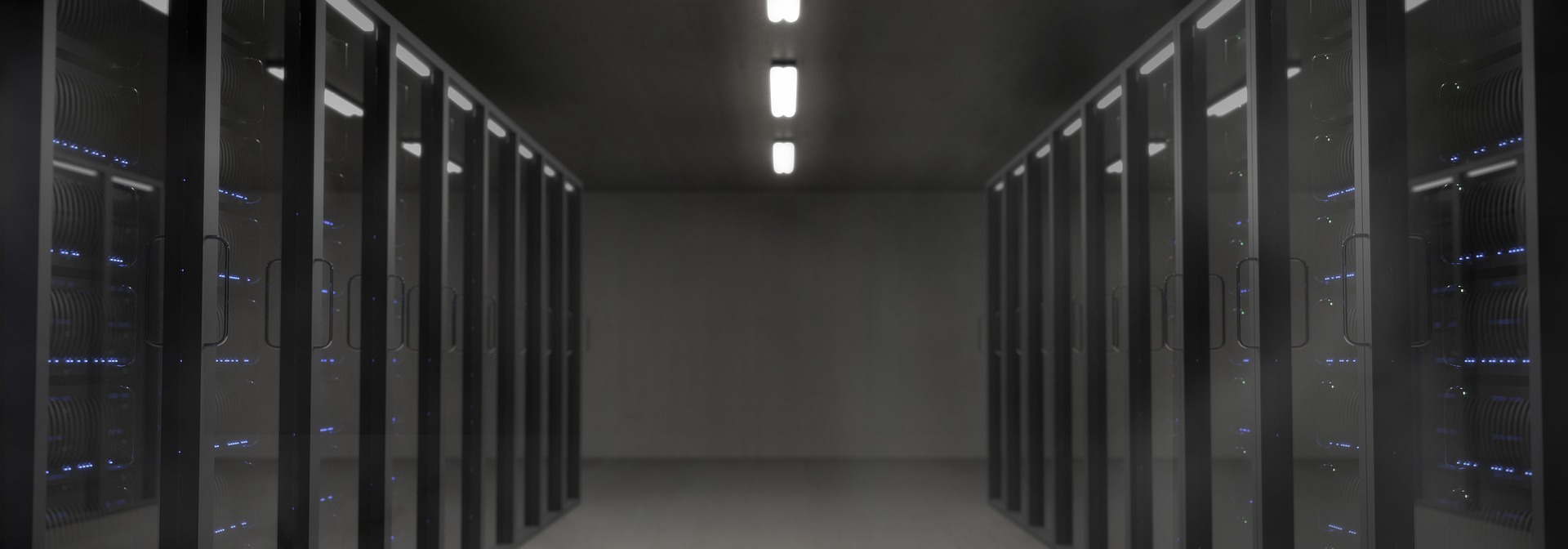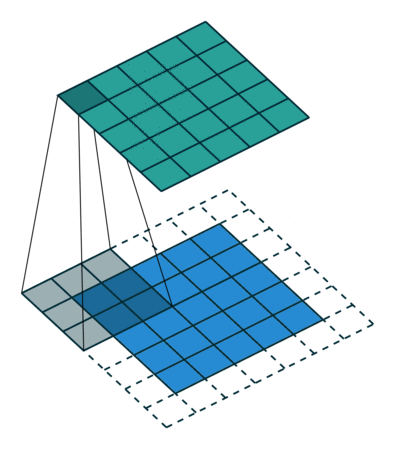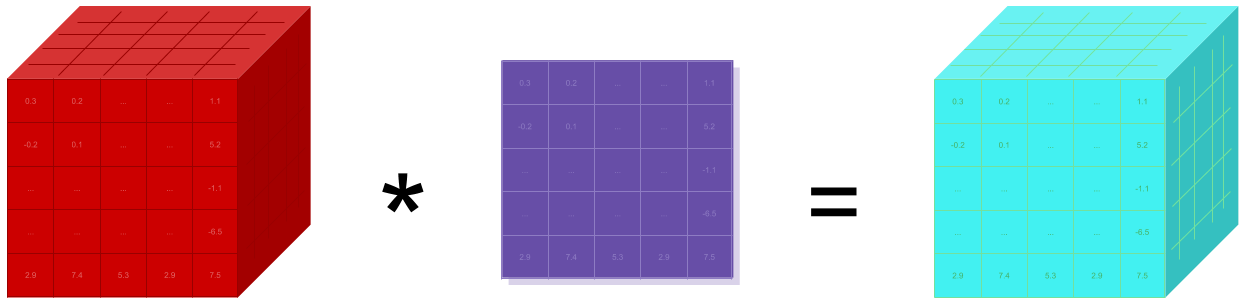# PyTorch - Python Deep Learning Neural Network API

Deep Learning Course - Level: Intermediate

## CUDA Explained - Why Deep Learning uses GPUs

### video

expand_more chevron_left

### text

expand_more chevron_left

### Why Deep Learning and Neural Networks uses GPUs

Welcome back to this series on neural network programming with PyTorch. In this post, we are going to introduce CUDA at a high-level.The goal of this post is to help beginners understand what CUDA is and how it fits in with PyTorch, and more importantly, why we even use GPUs in neural network programming anyway.

#### Graphics processing unit (GPU)

To understand CUDA, we need to have a working knowledge of graphics processing units (GPUs). A GPU is a processor that is good at handling specialized computations.

This is in contrast to a central processing unit (CPU), which is a processor that is good at handling general computations. CPUs are the processors that power most of the typical computations on our electronic devices.

A GPU can be much faster at computing than a CPU. However, this is not always the case. The speed of a GPU relative to a CPU depends on the type of computation being performed. The type of computation most suitable for a GPU is a computation that can be done in parallel.

#### Parallel computing

Parallel computing is a type of computation where by a particular computation is broken into independent smaller computations that can be carried out simultaneously. The resulting computations are then recombined, or synchronized, to form the result of the original larger computation.The number of tasks that a larger task can be broken into depends on the number of cores contained on a particular piece of hardware. Cores are the units that actually do the computation within a given processor, and CPUs typically have four, eight, or sixteen cores while GPUs have potentially thousands.

There are other technical specifications that matter, but this description is meant to drive the general idea.

With this working knowledge, we can conclude that parallel computing is done using GPUs, and we can also conclude that tasks which are best suited to be solved using a GPU are tasks that can be done in parallel. If a computation can be done in parallel, we can accelerate our computation using parallel programming approaches and GPUs.

### Neural networks are embarrassingly parallel

Let's turn our attention now to neural networks and see why GPUs are used so heavily in deep learning. We have just seen that GPUs are well suited for parallel computing, and this fact about GPUs is why deep learning uses them. Neural networks are embarrassingly parallel.

In parallel computing, an embarrassingly parallel task is one where little or no effort is needed to separate the overall task into a set of smaller tasks to be computed in parallel.

Tasks that embarrassingly parallel are ones where it's easy to see that the set of smaller tasks are independent with respect to each other.Neural networks are embarrassingly parallel for this reason. Many of the computations that we do with neural networks can be easily broken into smaller computations in such a way that the set of smaller computations do not depend on one another. One such example is a convolution.

#### Convolution example

Let's look at an example, the convolution operation:This animation showcases the convolution process without numbers. We have an input channel in blue on the bottom. A convolutional filter shaded on the bottom that is sliding across the input channel, and a green output channel:

• Blue (bottom) - Input channel
• Shaded (on top of blue) - `3 x 3` convolutional filter
• Green (top) - Output channel

For each position on the blue input channel, the `3 x 3` filter does a computation that maps the shaded part of the blue input channel to the corresponding shaded part of the green output channel.

In the animation, these computations are happening sequentially one after the other. However, each computation is independent from the others, meaning that none of the computations depend on the results of any of the other computations.

As a result of this, all of these independent computations can happen in parallel on a GPU and the overall output channel can be produced.

This allows us to see that the convolution operation can be accelerated by using a parallel programming approach and GPUs.

### Nvidia hardware (GPU) and software (CUDA)

This is where CUDA comes into the picture. Nvidia is a technology company that designs GPUs, and they have created CUDA as a software platform that pairs with their GPU hardware making it easier for developers to build software that accelerates computations using the parallel processing power of Nvidia GPUs.An Nvidia GPU is the hardware that enables parallel computations, while CUDA is a software layer that provides an API for developers.

As a result, you might have guessed that an Nvidia GPU is required to use CUDA, and CUDA can be downloaded and installed from Nvidia's website for free.

Developers use CUDA by downloading the CUDA toolkit. With the toolkit comes specialized libraries like cuDNN, the CUDA Deep Neural Network library.### PyTorch comes with CUDA

One of the benefits of using PyTorch, or any other neural network API is that parallelism comes baked into the API. This means that as neural network programmers, we can focus more on building neural networks and less on performance issues.

With PyTorch, CUDA comes baked in from the start. There are no additional downloads required. All we need is to have a supported Nvidia GPU, and we can leverage CUDA using PyTorch. We don't need to know how to use the CUDA API directly.

Now, if we wanted to work on the PyTorch core development team or write PyTorch extensions, it would probably be useful to know how to use CUDA directly.

After all, PyTorch is written in all of these:

• Python
• C++
• CUDA

### Using CUDA with PyTorch

Taking advantage of CUDA is extremely easy in PyTorch. If we want a particular computation to be performed on the GPU, we can instruct PyTorch to do so by calling `cuda()` on our data structures (tensors).

Suppose we have the following code:

```> t = torch.tensor([1,2,3])
> t
tensor([1, 2, 3])
```

The tensor object created in this way is on the CPU by default. As a result, any operations that we do using this tensor object will be carried out on the CPU.

Now, to move the tensor onto the GPU, we just write:

```> t = t.cuda()
> t
tensor([1, 2, 3], device='cuda:0')
```

This ability makes PyTorch very versatile because computations can be selectively carried out either on the CPU or on the GPU.

#### GPU can be slower than CPU

We said that we can selectively run our computations on the GPU or the CPU, but why not just run every computation on the GPU?

Isn't a GPU faster than a CPU?

The answer is that a GPU is only faster for particular (specialized) tasks. One issue that we can run into is bottlenecks that slow our performance. For example, moving data from the CPU to the GPU is costly, so in this case, the overall performance might be slower if the computation task is a simple one.Moving relatively small computational tasks to the GPU won't speed us up very much and may indeed slow us down. Remember, the GPU works well for tasks that can be broken into many smaller tasks, and if a compute task is already small, we won't have much to gain by moving the task to the GPU.

For this reason, it's often acceptable to simply use a CPU when just starting out, and as we tackle larger more complicated problems, begin using the GPU more heavily.

### GPGPU computing

In the beginning, the main tasks that were accelerated using GPUs were computer graphics. Hence the name graphics processing unit, but in recent years, many more varieties parallel tasks have emerged. One such task as we have seen is deep learning.

Deep learning along with many other scientific computing tasks that use parallel programming techniques are leading to a new type of programming model called GPGPU or general purpose GPU computing.

GPGPU computing is more commonly just called GPU computing or accelerated computing now that it's becoming more common to preform a wide variety of tasks on a GPU.

Nvidia has been a pioneer in this space. Nvidia refers to general purpose GPU computing as simply GPU computing. Nvidia's CEO Jensen Huang's has envisioned GPU computing very early on which is why CUDA was created nearly 10 years ago.

Even though CUDA has been around for a long time, it is just now beginning to really take flight, and Nvidia's work on CUDA up until now is why Nvidia is leading the way in terms of GPU computing for deep learning.

When we hear Jensen talk about the GPU computing stack, he is referring to the GPU as the hardware on the bottom, CUDA as the software architecture on top of the GPU, and finally libraries like cuDNN on top of CUDA.

This GPU computing stack is what supports general purpose computing capabilities on a chip that is otherwise very specialized. We often see stacks like this in computer science as technology is built in layers, just like neural networks.

Sitting on top of CUDA and cuDNN is PyTorch, which is the framework were we'll be working that ultimately supports applications on top.

This paper takes a deep dive into GPU computing and CUDA, but it goes much deeper than we need. We will be working near the top of the stack here with PyTorch.However, it's beneficial to have a birds eye view of just where we're operating within the overall stack.

### Tensors are up next

We are ready now to jump in with section two of this neural network programming series, which is all about tensors.We are ready now to jump in with section two of this neural network programming series, which is all about tensors. I hope you found this post useful. We should now have a good understand about why we use GPUs for neural network programming. For the first part of this series, we will be using a CPU. We are ready now to start working with `torch.Tensor`s and building our first neural networks.

I'll see you in the next one!

### quiz

expand_more chevron_leftDEEPLIZARD Message notifications

### resources

expand_more chevron_left

expand_more chevron_leftDEEPLIZARD Message notifications